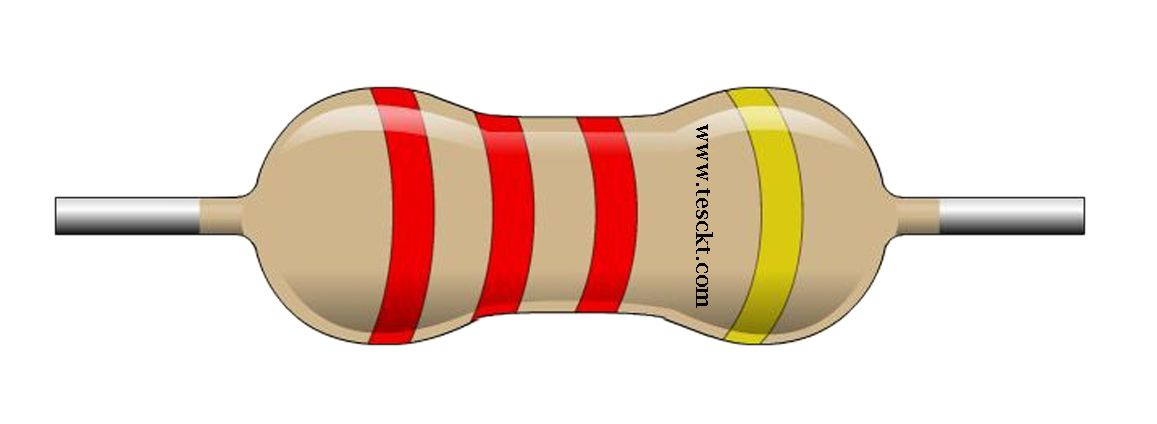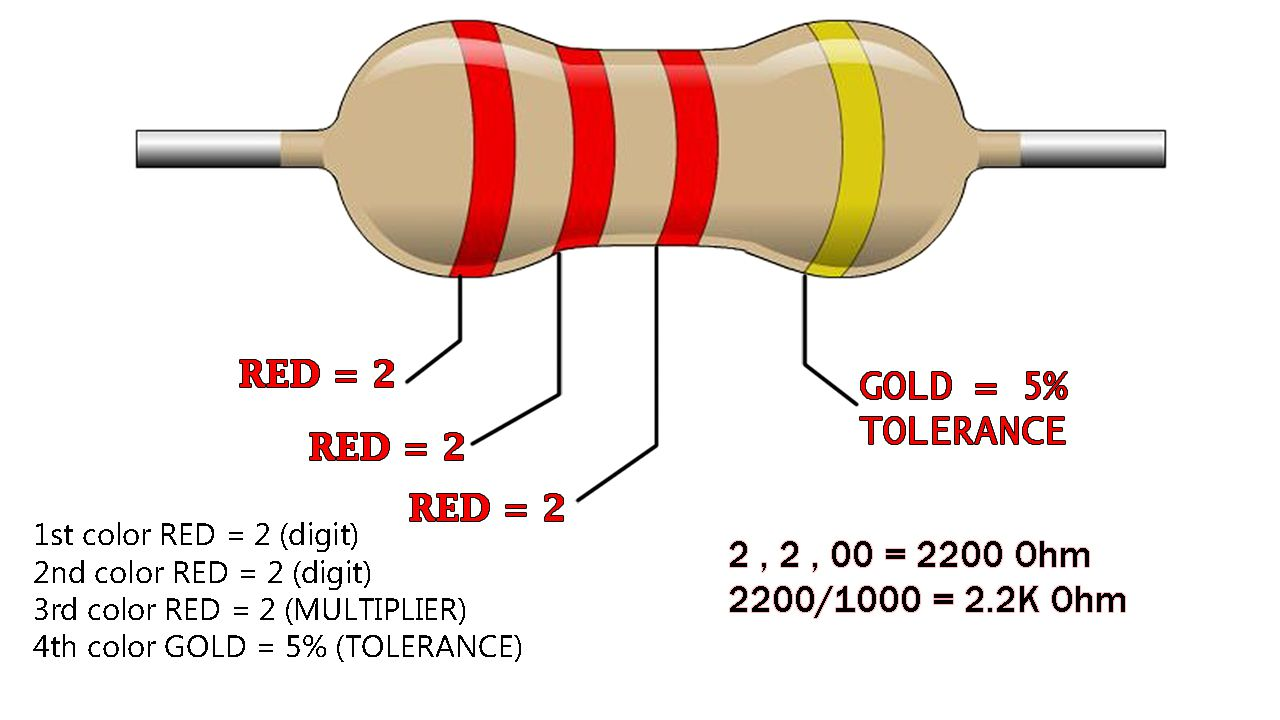## 2.2 k ohm resistor color code

2.2k resistor color code of the ¼ watt resistors are explained here, quicker resistor color coding is always a useful for every electronic engineer.2.2 k ohm resistor

2.2k
resistor color code of 4 band resistor, which is first 2 color bands is digits, 3rd color band is a multiplier and the last 4th band is tolerance band2.2 k ohm color code

1st color RED = 2 (digit)

2nd color RED = 2 (digit)

3rd color RED = 2 (MULTIPLIER)

4th color GOLD = 5% (TOLERANCE)

2.2 k resistors are the decimal resistance value, comparing with digit value, 2.2k is much specific.

First two RED bands are taken as digits

And Third band is multiplier

= 2-2-00/1000

= 2.2kohm 5%

This decimal value is produced when the multiplier value is divided by 1000 and also decimal come into play, then that zero make a decimal value between both twos.